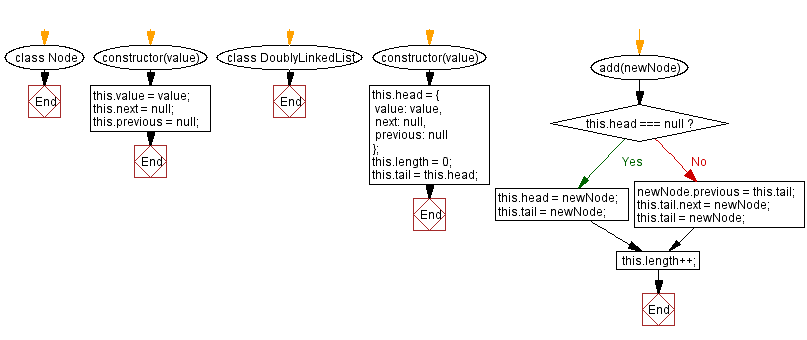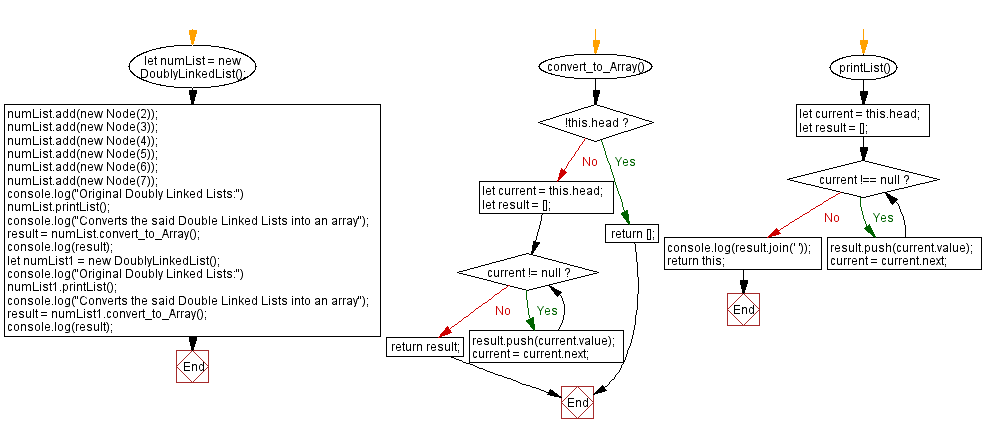# JavaScript Exercises: Convert a Doubly Linked lists into an array

## JavaScript Data Structures: Exercise-10 with Solution

Write a JavaScript program to convert a Doubly Linked lists into an array and returns it.

Sample Solution:

JavaScript Code:

``````class Node {
constructor(value) {
this.value = value;
this.next = null;
this.previous = null;
}
}
constructor(value) {
value: value,
next: null,
previous: null
};
this.length = 0;
}

this.tail = newNode;
}
else
{
newNode.previous = this.tail;
this.tail.next = newNode;
this.tail = newNode;
}

this.length++;
}

convert_to_Array() {
let result = [];
while (current != null) {
result.push(current.value);
current = current.next;
}
return result;
}

printList() {
let result = [];
while (current !== null) {
result.push(current.value);
current = current.next;
}
console.log(result.join(' '));
return this;
}
}

numList.printList();
console.log("Converts the said Double Linked Lists into an array");
result = numList.convert_to_Array();
console.log(result);
numList1.printList();
console.log("Converts the said Double Linked Lists into an array");
result = numList1.convert_to_Array();
console.log(result);
```
```

Sample Output:

```Original Doubly Linked Lists:
2 3 4 5 6 7
Converts the said Double Linked Lists into an array
[null,2,3,4,5,6,7]
""
Converts the said Double Linked Lists into an array
[null]
```

Flowchart:Live Demo:

See the Pen javascript-doubly-linked-list-exercise-10 by w3resource (@w3resource) on CodePen.

Improve this sample solution and post your code through Disqus

What is the difficulty level of this exercise?

Test your Programming skills with w3resource's quiz.

﻿

## JavaScript: Tips of the Day

Returns the average of an array, after mapping each element to a value using the provided function

Example:

```const averageBy = (arr, fn) =>
arr.map(typeof fn === 'function' ? fn : val => val[fn]).reduce((acc, val) => acc + val, 0) /
arr.length;

console.log(averageBy([{ n: 2 }, { n: 4 }, { n: 6 }, { n: 8 }], o => o.n));
console.log(averageBy([{ n: 1 }, { n: 3 }, { n: 5 }, { n: 7 }], 'n'));
```

Output:

```5
4
```